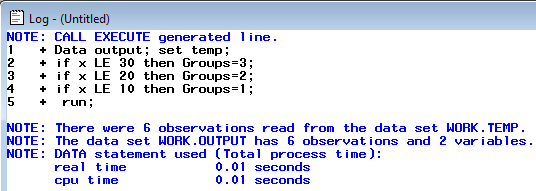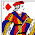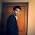# SAS : Call Execute Made Easy

This tutorial explains how to use data step to interact with the SAS macro facility.

Example 1
Suppose you have two data sets named "temp" and "temp2". You are asked to form a group based on the logical conditions given in the other dataset "temp2" and apply the conditions in dataset temp.

Use columns VAR, SCORE and RANK of dataset TEMP2 to create the following conditions and apply them in dataset TEMP-
if x less than or equal to 30 then Groups=3;
if x less than or equal to 20 then Groups=2;
if x less than or equal to 10 then Groups=1;

Create sample data sets
Data temp;
input x;
cards;
5
10
15
20
25
30
;
run;
Data temp2;
input var \$ Score rank Section \$;
cards;
x 30 3 C
x 20 2 B
x 10 1 A
;
run;
Solution : CALL EXECUTE
Data _null_;
set temp2 end=last;
if _n_=1 then call execute ('Data output; set temp;');
call execute ("if " ||strip(Var)|| " LE " ||strip(Score) || " then " || "Groups" || "=" ||strip(rank) ||";");
if last then call execute (' run;');
run;
Note - All static components should in single or double quotes  and variable components should not be in quotes.Log : CALL EXECUTE

Form a new Character variable using the following conditions :
If x LE 30 then Class="C";
If x LE 20 then Class="B";
If x LE 10 then Class="A";

Solution : CALL EXECUTE
Data _null_;
set temp2 end=last;
if _n_=1 then call execute ('Data output; set temp; length Class \$10.;');
call execute ("if " ||strip(Var)|| " LE " ||strip(Score) || " then " || "Class" ||  "=" || """" || strip(Section) ||""""|| ";");
if last then call execute (' run;');
run;
Example 2 : Print Multiple Datasets
data _null_ ;
input mydata \$char50. ;
call execute
("proc print" || " data = " || strip(mydata) || ";" || "run ;");
cards;
temp
temp2
output
run;
The above program prints 3 datasets - temp temp2 output.

Example 3 : Call a Macro
%macro mymacro(k);
data want;
set temp;
%do i = 1 %to &k;
if _N_ = &i then y = %eval(&i.* 10);
%end;
run;
%mend;
data _null_;
call execute ('%mymacro(6)');run;
Example 4 : Dynamically Call Macro
%macro mymacro(i,j);
%put first = &i. second = &j.;
%mend;
DATA example;
input x y \$32. ;
datalines;
1 temp
2 temp2
;
run;
data _null_;
set example;
call execute('%MyMacro('||x||','||y||')');
run;Deepanshu founded ListenData with a simple objective - Make analytics easy to understand and follow. He has over 8 years of experience in data science. During his tenure, he has worked with global clients in various domains like Banking, Insurance, Telecom and Human Resource.

Related Posts:
6 Responses to "SAS : Call Execute Made Easy"
1.Fantastic tutorials and blog. I am preparing for Base SAS certification exam so I am learning different topics from your blog. I was exhausted when I found. This is very completed and amazing.

1.Thank you for your kind words. Wish you all the best for your Base SAS certification exam.

2.what is the purpose for the call execute?

3.I was thinking of making video tutorials with simple examples. Your blog is really great in the reading format. Simple examples are the key to quickly understand concepts. Good luck. Thank you.

4.5.